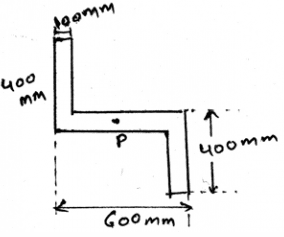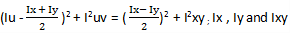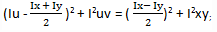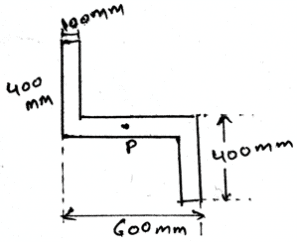# Test: Mohr’s Circle For Moments Of Inertia

## 15 Questions MCQ Test Engineering Mechanics | Test: Mohr’s Circle For Moments Of Inertia

Description
This mock test of Test: Mohr’s Circle For Moments Of Inertia for Mechanical Engineering helps you for every Mechanical Engineering entrance exam. This contains 15 Multiple Choice Questions for Mechanical Engineering Test: Mohr’s Circle For Moments Of Inertia (mcq) to study with solutions a complete question bank. The solved questions answers in this Test: Mohr’s Circle For Moments Of Inertia quiz give you a good mix of easy questions and tough questions. Mechanical Engineering students definitely take this Test: Mohr’s Circle For Moments Of Inertia exercise for a better result in the exam. You can find other Test: Mohr’s Circle For Moments Of Inertia extra questions, long questions & short questions for Mechanical Engineering on EduRev as well by searching above.
QUESTION: 1

### Which of the following is the equation which we get for the graphical solution of the moment of inertia?

Solution:

In the graphical method of solving the moment of inertia of the structures, we have the answer as the driven equation. Thus this equation is used so as to ease the calculations. This equation is derived from the equations of the product of area inertia. Thus the equation.

QUESTION: 2

### What is the radius of the Mohr circle of the structure given below.Solution:

In the above question, Ix , Iy and Ixy are meant to be calculated. This is because they are the derived quantity. It is dependent on the values of the structure which is constant. It means they are derived from constant values of the structure, thus they becomes constant value.

QUESTION: 3

### If a car is moving forward, what is the direction of the moment of the moment caused by the rotation of the tires?

Solution:

When you curl your wrist in the direction in which the tires are moving then you will find that the thumb is pointing outwards. That is outwards the body of the car. This phenomena is also observed in rainy seasons. When cars travel on the roads, the water is thrown outside from the tires, due to moment.

QUESTION: 4

The body is sometimes acted by two or three force members and we need to find the Mohr‘s circle for inertia for the same. The difference between the two and the three force members is:

Solution:

The definition of the two force member only defines that the forces are being acted on the two points on the body. So does is the definition of the three forces members. The points of action of the three forces are three.

QUESTION: 5

Which of the following is the equation which we get for the graphical solution of the moment of inertia?

Solution:

In the graphical method of solving the moment of inertia of the structures, we have the answer as the driven equation. Thus this equation is used so as to ease the calculations. This equation is derived from the equations of the product of area inertia. Thus the equation.

QUESTION: 6

In the equationSolution:

In the above equation, Ix , Iy and Ixy are constants. This is because they are the derived quantity. It is dependent on the values of the structure which is constant. It means they are derived from constant values of the structure, thus they are constant value.

QUESTION: 7

Whenever the distributed loading acts perpendicular to an area its intensity varies __________ for the determination of the Mohr’s circle for inertia.

Solution:

The load intensity is varying linearly in the structures. Thus the intensity is not varying parabolically nor is it cubically. It cannot be a vector also. Thus the intensity is linearly varied.

QUESTION: 8

State whether true or false.this equation is wrong for the calculations of the moments.

Solution:

The above equation is true for the calculation of the moments. , here Ix , Iy and Ixy are constants. This is because they are the derived quantity from the constants itself. This is the graphical method of solving the moment of inertia of the structures.

QUESTION: 9

What is the Imax of the Mohr circle of the structure given below?Solution:

In the above question, Ix , Iy and Ixy are meant to be calculated. This is because they are the derived quantity. It is dependent on the values of the structure which is constant. It means they are derived from constant values of the structure, thus they becomes constant value.

QUESTION: 10

What does the moment of the force measure in the calculation of the Mohr circle’s data for the moments of inertia?

Solution:

The moment of the force measures the tendency of the rotation of the body along any axis, whether it be the centroid axis of the body, or any of the outside axis. The couple moment is produced by two forces, not by a single force. The total work done is the dot product of force and distance not the cross. A use of Mohr circle can also help in determining the direction.

QUESTION: 11

State whether true or false. (Iu – a)2 + I2uv = R2; this equation is wrong for the calculations of the moments.

Solution:

The above equation is true for the calculation of the moments. Here Iu and Iuv are not constants. This is because they are the derived quantity from the variables. This is the graphical method of solving the moment of inertia of the structures.

QUESTION: 12

The Mohr’s circle for an area is helpful so as to________

Solution:

The Mohr’s circle is important as to know what is the maximum and the minimum moment of inertia of an area. This data is required as to design the structure of the body. This means that the designing of the body is majorly done by the help of the determination of the product of the moment of Inertia.

QUESTION: 13

If the non-Uniform loading is of the type of parabola then for calculating the Mohr’s circle for the inertia for areas?

Solution:

The net force will act at the centroid of the parabola. Whether it be a parabola or the cubic curve the centroid is the only point at which the net force act. Force can’t be acted horizontally if the loading is vertical. Hence whatever be the shape of the loading, the centroid is the point of action of net force. Thus the use of centroid.

QUESTION: 14

If any external force also is applied on the structure and we are determining the Mohr’s circle for the inertia then what should we consider?

Solution:

The external forces are treated differently. They are not added by the force of the distributed loading. That is the force not only acts at the centroid always. It can be shifted also. Depending on the external forces. Thus the use of centroid or centre of mass.

QUESTION: 15

The tendency of rotation of the body along any axis is also called ___________. As used for the construction of the Mohr circle.

Solution:

The tendency of rotation of the body along any axis also called the torque. It is the moment of the force acting perpendicular to the direction of the axis of rotation. If the axis and the force are meeting at any point then there is no moment applied by the force.